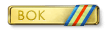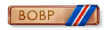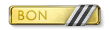# Yak_Panther

Members

32

## Community Reputation

49 Excellent

• Rank
Member
•## Recent Profile Visitors

470 profile views

• ### theRedPanda

1. Using a weight of 5600 lbs*3, a wing area of 174 ft^2, a density = 12k ft, .84 kg/m^3, at 192 mph, I get a Cl of 1.494. What variables are you using?
2. The definition of a sustained turn is; a turn that does not require the energy state of the aircraft to change for the given turn rate / load factor to be maintained indefinitely. The energy state being defined by the speed, and altitude of the aircraft, Pe, ke. If the aircraft has to descend to maintain a given turn rate / load factor, Then that turn is not sustainable. If the aircraft is capable of climbing during a given turn, That indicates, that the aircraft has excess power and is capable of sustaining that turn. In Gates's chart, turns at load factors and airspeed below the
• 139 replies
• 1
•3. Any turn made below the zero angle of straight climb line, is sustained. Turns above the line require the craft lose alt. See gates paper or the notes at the bottom of the test. Guess where the 2 g point is?
4. That's not the minum turn radius at 2 g. That was a Test of the 2 g sustained turn at 12k feet. If you wanted to test the minimum radius, you would pull 2 g and reduce the throttle until you could you could no longer fly at 2g. I showed how those were computed. Gates gives the Stall Boundary line as: n (load factor) = N Where N is (V/Vs)^2 At the 2g where the TAS is 150mph the equation looks like this. 2= (150/Vs)^2 All they did was Solve Vs as Vs = 75 * Sqrt2 Vs = 106.07 TAS. Then the went and plotted the radius and the rest of points
5. Does the aircraft have excess power in the 2 G min sustained rate speed?, yes other wise this would be the corner speed. Would Going slower or faster cause the radius to shrink? What is limiting factor of low speed flight?
6. Max rate sustained is power limited. Min radius sustained is load factor limited.
7. Fig 17 is not based on table 4. It's Kludged from table 6. The point is at 150mph because, they took ASI in chart 6, 118 mph: , and using the equation at the bottom of the chart. converted it to TAS 147: Then, based on weight and wing area computed the Cl Max at 2g, 1.6 The Rest of the CL's were computed by taking the approximate Delta in Cl due G of Spit and applying it to 109. Which is how you get a 109 with a 1g Cl max of 1.95 The only flight test conducted to devise these charts were a 2 g min turn radi
• 139 replies
• 1
•8. Vi corrected is 129 CAS. TAS is = 161. Pilots ASI is 118 CAS; TAS is =147. The chart shows the conversion from TAS to CAS as Vi= V*Sqrt of sigma. Where sigma is Pressure alt / Pressure sea level in Lbf^3. Sigma =.053/.076 ~ .7 sqrt of .7 = .8 Vi = V * .8 Therefor: Vi / .8 = V Vi 118/ .8 ~ 147 TAS. The chart, Fig 17, is based off the Pilots ASI. Not the corrected indicated airspeed, CAS 129, from the trailing probe. If you compute the 2g stall speed at the conditions provide in the chart with the corrected CAS converted to TAS, 161 mph. The C
9. If DCS is benchmark for accuracy for the 109. Then testing has already has proven that Il 2 is accurate, ie very close to the DCS 109 K4. This show the specific excess power of both the DCS and Il 2 K-4 1.8 ata @ sea level are equal. Sustained turn rate is defined by Specific Excess Power (Ps) is = (Trust-Drag)*V/Weight. The Sustained Rate line in these E-M charts is the Ps-0 line. That is 0 = (Trust-Drag)*V/Weight Since both of these curves match, we can conclude that both aircraft have the same total amount thrust and drag, including induced. The in
• 139 replies
• 8
•10. I don't think the heating system is modeled, there doesn't seem to any indication of it in the file structure. The best way to test the effect of temperature on the the effects of dispersion is. To conduct the testing on the ground at the same runway, then vary the season in the editor to change the temps. IE Going from winter to summer. I like to use The P-39 here because the 50 cals guns are basically level, due to the tricycle style landing gear. There's also only two guns, so its a bit easier to observe the rounds. Ground testing also minimizes the recoil effects, since the gu
• 198 replies
• 1
•11. How did you decide that 0.1 was the correct amount? I think you may find that your pattern is likely to change based on the map's ambient temperature. Also, how are you accounting for distance in your measurements? If your measuring 10 cm of dispersion at 100m, then dispersion is 1 mil. However, if you measure 10cm of dispersion at 200m, then the dispersion is .5 mil. Based on your P-47 example (4 mil 100%) at 610 meters all the rounds should fall with in 2.4 meters of the aim point. Then you also have to account for convergence. I'm just wondering how you account for those va
• 198 replies
• 1
•• 131 replies
• 2
•13. Literally never said that. WW2 aircraft wings are cambered. Even during level flight the angle of attack is greater than 1 degree, So the chances of rounds impact at 90 degrees are not very common.
• 901 replies
• 1
•14. If we look at the 70 degree ricochet figure 2-34 pg 63/49 from the AGARD report. You can determine that the ricochet is a result of the impact velocity not impact angle alone. The impact velocity is noted on that sheet of aluminum in the pic, 1100 fps. The document gives the formula to compute the critical velocity to perforate plates at varying degrees of obliquity. Page 51/ 37. It’s the ballistic limit formula used throughout the terminal ballistics. V50 = {C [Pc (Density of core)* t (Target Thickness)*Ap(presented area of projectile);/ W (Weight of the projectile)]^B(E
• 901 replies
• 7
•×
×
• Create New...